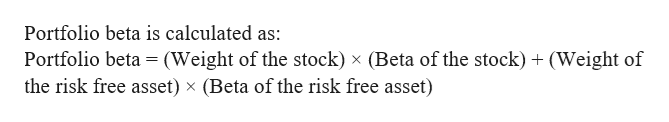# An investor has a portfolio of two securities, a stock with a beta of 1.24 and a risk-free asset. The stock has an expected return of 13.68% and the risk-free asset has an expected return of 2.80%. The portfolio beta is 0.65. What rate of return should the investor expect to earn on her portfolio? Group of answer choices8.50%9.16%9.33%9.41%

Question
36 views
An investor has a portfolio of two securities, a stock with a beta of 1.24 and a risk-free asset. The stock has an expected return of 13.68% and the risk-free asset has an expected return of 2.80%. The portfolio beta is 0.65. What rate of return should the investor expect to earn on her portfolio?

8.50%
9.16%
9.33%
9.41%
check_circle

Step 1

Suppose the weight of the stock in the portfolio be x, then the weight of the risk free asset in the portfolio will be 1-x.

Step 2help_outlineImage TranscriptionclosePortfolio beta is calculated as: Portfolio beta = (Weight of the stock) x (Beta of the stock)(Weight of the risk free asset) x (Beta of the risk free asset) fullscreen
Step 3

The beta of a risk free asset will be zero.
Given that portfolio beta is 0...

### Want to see the full answer?

See Solution

#### Want to see this answer and more?

Solutions are written by subject experts who are available 24/7. Questions are typically answered within 1 hour.*

See Solution
*Response times may vary by subject and question.
Tagged in